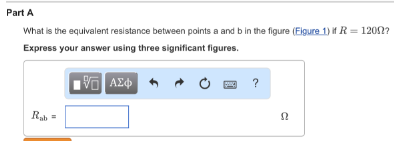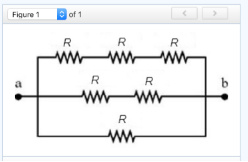# What is the equivalent resistance between points a and b in the figure (Figure 1) if R=120?

What is the equivalent resistance between points a and b in the figure (Figure 1) if R=120??